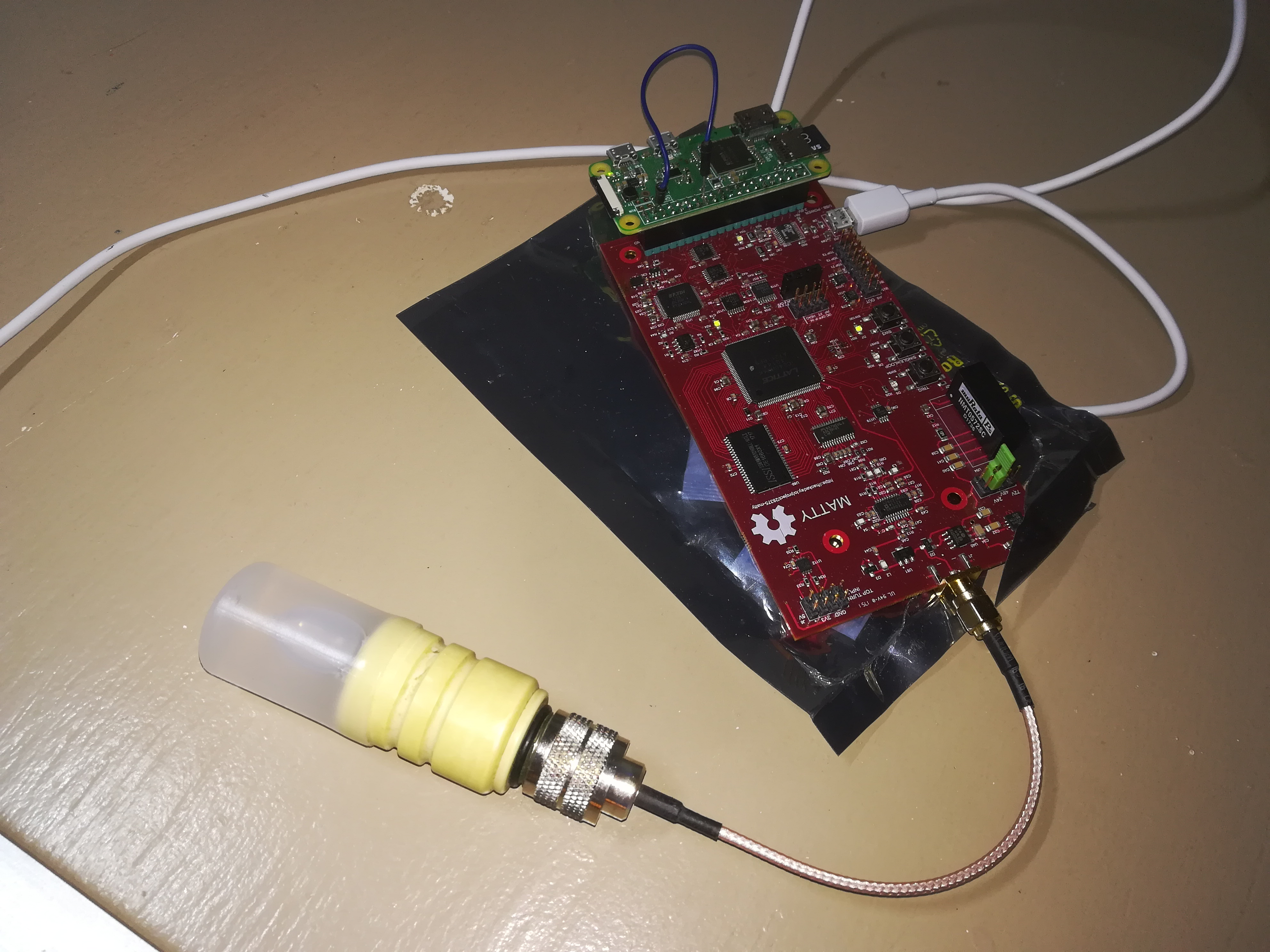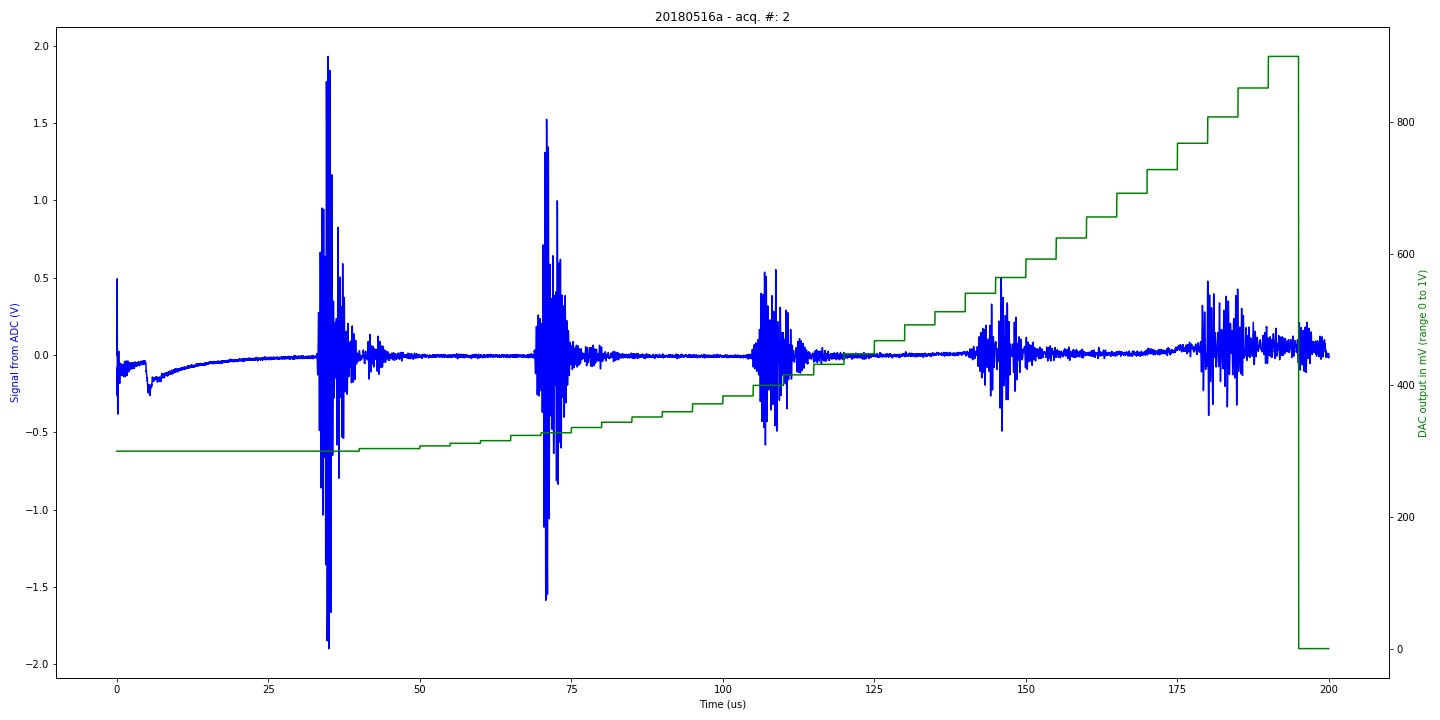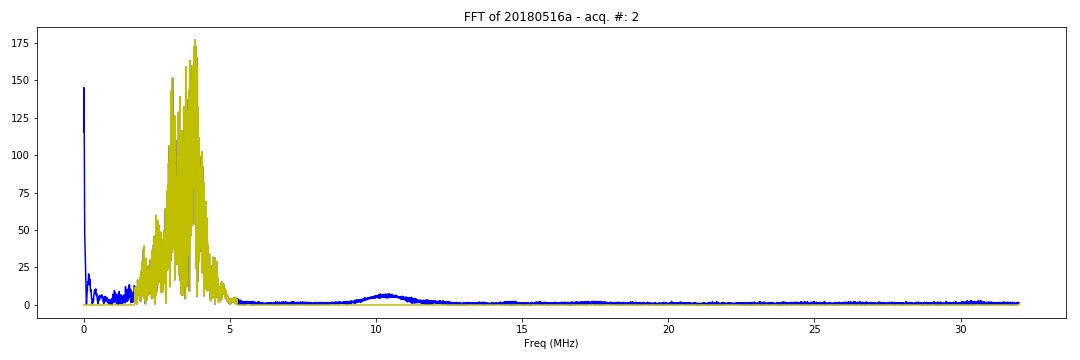# How to manage an experiment.

That's a review of an existing experiment using the pyUn0 v1.0.

All experiments (on this hardware and other) are on this repo.

## Setup

All calibration / basic experiments were using a piezo with a reflector a few cm away, with water in between.

I used in this case a film case, just right for the size of the transducer, filed with water and connected to the board.## Acquisition

### Imports

Let's start by importing

``````#!/usr/bin/python
import spidev
import RPi.GPIO as GPIO
import time
import numpy as np
import matplotlib
matplotlib.use('Agg')
import matplotlib.pyplot as plt
import json
import time
from pyUn0 import *
``````

### Setup

And now we setup the acquisition. In particular, setting up the firmware info and empty arrays.

``````x = us_spi()
x.JSON = {}
x.JSON["firmware_version"]="e_un0"
x.JSON["data"]=[]
x.JSON["registers"]={}
x.JSON["parameters"]={}
x.JSON["experiment"]={}
x.JSON["experiment"]["id"] = "20180721a"
x.JSON["experiment"]["description"]="Classical experiment with calibration piezo"
x.JSON["experiment"]["target"] = "calibration rig"
x.JSON["experiment"]["position"] = "0"
x.JSON["V"]="25"

x.StartUp()
x.ConfigSPI()

# Setting acquition speed
f = 0x00
x.WriteFPGA(0xED,f) # Frequency of ADC acquisition / sEEADC_freq (3 = 16Msps, 1 = 32, 0 = 64, 2 = 21Msps)

x.WriteFPGA(0xEB,0x00) # Doing one line if 0, several if 1
x.WriteFPGA(0xEC,0x01) # Doing 1 lines
if x.JSON["registers"]: # means it's set to 1, ie that's multiples lines
NLines = x.JSON["registers"]
else:
NLines = 1

Fech = int(64/((1+f)))
``````

### Pulses profiles

Then we setup the pulse train:

• A pulse of 200ns
• Damping for 2us
• Start of the acquisition 3us after the pulses
• Acquisition for 200us
``````
x.JSON["N"] = 1 # Experiment ID

# Timings
t1 = 200
t2 = 100
t3 = 2000
t4 = 3000-t1-t2-t3
t5 = 200000

LAcq = t5/1000 #ns to us
Nacq = LAcq * Fech * NLines

# Setting up the DAC, from 50mV to 850mv
Curve = x.CreateDACCurve(5,85,True)
x.setDACCurve(Curve)
# Setting pulses
x.setPulseTrain(t1,t2,t3,t4,t5)
``````

### Acquisition and file save

And then we proceed to acquisition itself. We reset the memory counter, trig, and then copy the acquisition into memory

``````# Trigger
x.WriteFPGA(0xEF,0x01) # Cleaning memory pointer
x.WriteFPGA(0xEA,0x01) # Software Trig : As to be clear by software

A = []
for i in range(2*Nacq+1):
A.append ( x.spi.xfer([0x00] ) )
a = np.asarray(A).astype(np.int16)

x.JSON["data"] = A

with open("acquisition.json", 'w') as outfile:
json.dump(x.JSON, outfile)
``````

That's it ;)

## Processing

### Basic processing of an acquisition file

``````x = us_json()
x.JSONprocessing("acquisition.json") # to process the image
x.mkImg() # creates the image of the acquisition
x.PlotDetail(0,100,125) # plots the detail between 100us and 125us
x.SaveNPZ() # saves the data
``````### Other utilities

There are some other utilities.. to be enhanced ?

``````x.mkFFT()
``````